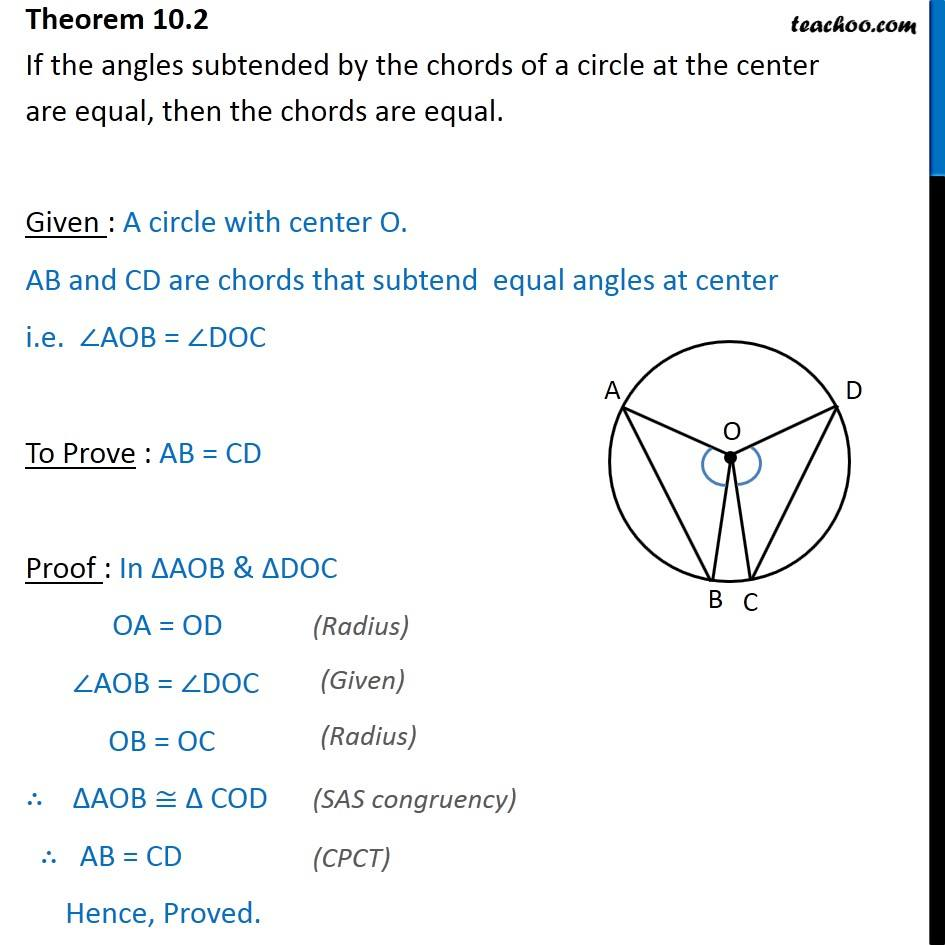Theorems

Chapter 9 Class 9 Circles
Serial order wiseLearn in your speed, with individual attention - Teachoo Maths 1-on-1 Class

### Transcript

Theorem 9.2 If the angles subtended by the chords of a circle at the center are equal, then the chords are equal. Given : A circle with center O. AB and CD are chords that subtend equal angles at center i.e. ∠AOB = ∠DOC To Prove : AB = CD Proof : In ΔAOB & ΔDOC OA = OD ∠AOB = ∠DOC OB = OC ∴ ∆AOB ≅ ∆ COD ∴ AB = CD Hence, Proved.